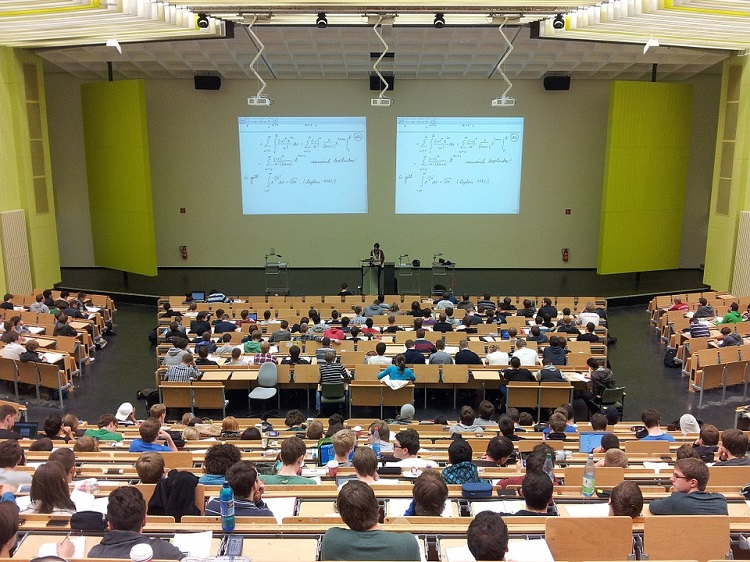# A Study on Graduate record examinationGRE refers to graduate record examination. It tests the individual on 3 bases: verbal reasoning, quantitative reasoning and analytical writing skills. It is roughly 4 hours. It is basically for people who aspire to apply for a masters course in foreign nations or for PhD applicants. This is a computer-based test. The bases followed are with a reason that it becomes easier for universities to compare people from various different backgrounds while giving seats. Each section includes 20 questions and 35 minutes is allotted for completion. Usually the scores range from 130-170.

GRE quant questions are basically done to check normal mathematical abilities and to get an insight of normal knowledge of concepts taught in previous classes. It also deals with ability to deal with various issues. Some topics are relied upon in real life situations while some are formulated on mathematical issues. Arithmetic topics include basic calculations like prime numbers, divisibility, properties and types of integers, factorization, arithmetic operations, percentages, ratios, absolute values, number lines, decimal representations and sequencing of numbers. Algebra topics include simplifying and factoring algebraic expressions, exponents, relations, inequalities, functions, solving linear and quadratic equations, setting and solving equations to world problems, geometry, graphs, intercepts and slopes of lines. Geometry includes parallel and perpendicular lines, triangles including isosceles, 30°-60°-90°, equilateral triangles, circles, polygons, quadrilaterals, similar and concurrent figures, 3-d figures, area and perimeters, volume, Pythagoras theorem and abilities to measure angels are also checked. Data analysis involves statistical tools such as mean, median, mode, standard deviation, range, percentiles, quartiles, interquartile ranges, interpretation of graphs and tables, line graphs, bar graphs, circle graphs, box plots, scatter diagrams, probabilities of compound events, elementary probability, independent and dependent events, random and conditional probabilities, counting methods like venn diagrams, permutations and combinations. There are no tests of inferential statistics. It also does not include trigonometry and higher-level calculus.

Terminologies and symbols are same like used in school level. Numbers which are used are real numbers. Figures such as circle and square and not drawn to scale but coordinate systems are drawn to scale. Graphical representations are also drawn to scale. These involve 4 types of questions:

1. Comparison of quantities:  quantities are compared and best suited options are selected.
2. Select one option choice: in this only 1 answer is to be selected from options available.
3. Select one or more options:  in these candidates have to choose one or more options from given options. The question may or may not specify the number to be chosen.
4. Numeral entry questions: in this the candidate has to fill answer in the form of numbers, fractions, decimals, etc. This is a computer operated test so use of mouse and keyboard is necessary.

All the problems of data interpretation are based on the tables and bar graphs presented along with the data given. Different and various kinds of strategies are to be used in case of problem-solving questions. Use of a basic calculator is allowed and since the test is computer based the on-screen calculator is always available for help. Different coaching classes are available for preparation of GRE quant questions.